# Catch up on the latest AI articles# StackVAE-G To Become A Powerful Gear, Cutting Into Multivariate Time Series Anomaly Detection

3 main points
✔️ Further proposed framework that takes into account the characteristics and objectives of multivariate time series data
✔️ Stacked block processing based on VAEs and reduced computational load
✔️ Improved performance and explainability by incorporating a graph learning module

## first of all

Regarding multivariate time-series anomaly detection, we have described in a previous article ( MTAD-GAT using graph-attention for multivariate time-series anomaly detection ), we have shown that the model performance can be improved by capturing not only the characteristics of individual time series data on the time axis but also the correlation among them.

The StackVAE-G presented here also follows this concept. There is no direct comparison with MTAD-GAT, perhaps because of the close timing of its presentation. MTAD-GAT mainly uses graph attention to capture the correlation between feature values and time axis correlation, and FCN and VAE are used as a prediction model and recovery model, respectively. (not attention) is used, which is quite different. I would like to take a look at this area as well.

This data example is the spacecraft data SMAP (Soil Moisture Active Passive satellite)collected by NASA, which was also used in the MTAG-GAT evaluation. We can see similarities and correlations between some of the data series.

##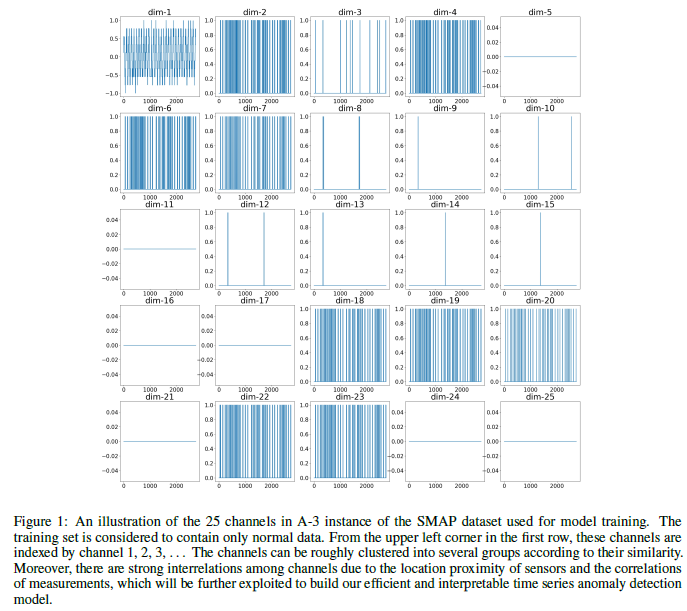These relationships can be seen in other time-series data, such as maintenance timing decisions, intrusion detection, fraud detection, disease outbreak detection, and AI operations. To perform effective anomaly detection, it is important to keep the following two points in mind

• reconstructive modeling
• correlation structure

For restoration modeling, we propose a stacked block-like restoration framework. The block-like VAE model is stacked with shared channel-level weights to apply to multivariate data. To extract the correlation structure between series, we use a graph learning module, in which each channel of the time series data corresponds to a node, to understand the correlation between distant points in time.

## background

### time-series anomaly detection

There are two types of unsupervised learning based on neural networks: prediction-based methods and restoration-based methods. An example of prediction-based methods is LSTM-NTD, while deep generative models are widely applied to restoration-based methods.

### Deep Generative Model (DGM)

Deep Generative Model (DGM) recovers the normal pattern of data input and detects the anomaly by the difference between the recovered model and the unsupervised one. (Figure1 (a), (b))

In the staged method, RNNs are used to build a recovery model of the data at each point in time; LSTM Encoder-Decoder, LSTM-VAE, GGM-VAE, OmniAnomaly, etc. have this structure. However, it has a weakness in that it tends to overtrain both normal and abnormal data. This is due to the strategy of building a recovery model for each time point.

To solve this problem, block-based methods use sliding windows to build a recovery model for each block, such as Donut and USAD. However, the size of the model weights is large, and more computing power and data are required to improve the learning accuracy. In the case of multivariate time series, it becomes more serious.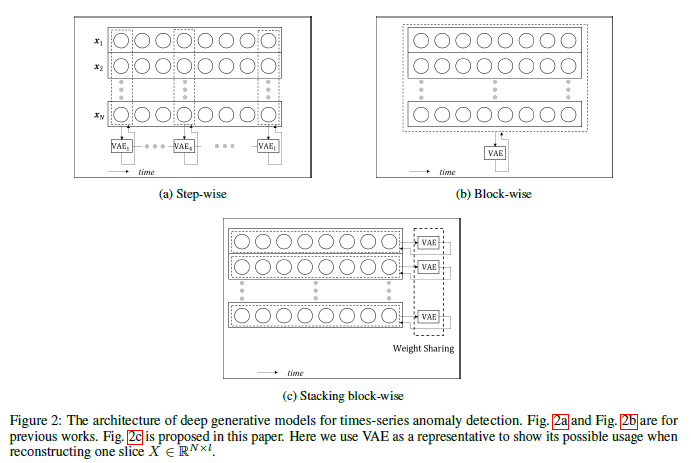### Graph Learning, Structure Learning

For multivariate time series data, autoregressive methods with endogenous variables can be used to construct vector autoregressive models. These recursive deep models contain endogenous correlations between channels and lack explanatory power.

## technique

The structure of StackVAE-G is as shown in Figure 3. The skeleton is a VAE, and the blue part is the Encoder and the pink part is the Decoder. The upper left block of the blue part is the graph learning model. It compares the upper time-series input and the lower time-series recovered data to determine the anomaly.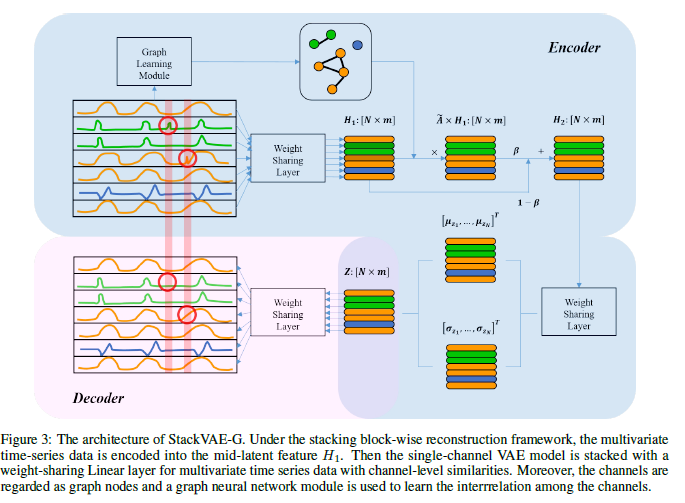### Laminated Block VAE Model

The stacked block VAE restoration model builds a single-channel block restoration and stacks it multiple times using weight sharing. (Figure 2c) The rows in the figure correspond to channels. The blockwise encoded latent variable $H_1$ is m-dimensional and of size nxm with n number of channels. In the output $\tilde{A}$ of the graph learning module described next, it is transformed into the latent variable $H_2$ in the second stage by weighting is as follows.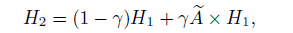The latent variable $H_2$ is passed through the decoder to obtain the recovered data.

### Graph Learning Module

The correlation structure between multiple channels of time series data is learned by an undirected graph network represented by the following equation.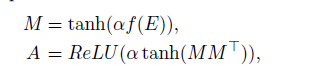$E$ is a node initialized with a random number, corresponding to a channel. The model is focused on the anomaly detection task and aims to learn time-invariant graphs of stable correlations under normal conditions in a probabilistic VAE framework.

We obtain $\tilde{A}$ by the following process that sets the other top k's with strong correlations to zero.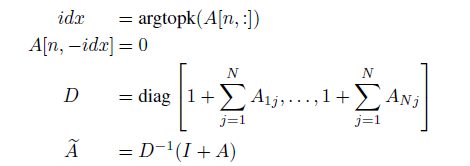### loss function

The loss function is the sum of the loss of the graph network and the loss of the VAE, as follows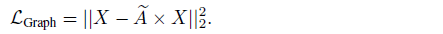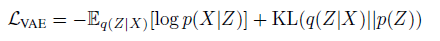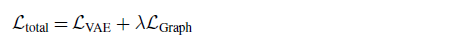### Learning and detection

For training, we combine two modules, the StackVAE model and the graph learning module, and optimize $L_{total}$ with Adam; we train an encoder consisting of the StackVAE and graph learning modules to infer the posterior latent distribution p(X|Z). Randomly sample from the approximate posterior distribution q(Z|X) and train the decoder to produce accurately recovered output.

For detection, with the normal pattern $\hat{X}$ recovered for input X, the heteroskedasticity score at each time t of X is expressed as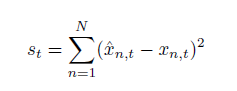## experiment

The data used for the evaluation are the NASA data SMAP, furthermore MSL (Mars Science Laboratory), and SMD (Server Machine Dataset).

The comparison is shown in Table 1. Most of them are presented in the previous article; IF (Isolation Forest) is a tree-structured ensemble that classifies variants efficiently [19 ]; USAD (Unsupervised Anomaly Detection) is a GAN-like adversarial learning model using two autoencoders that share an encoder . USAD (Unsupervised Anomaly Detection) is a model that performs adversarial learning like GAN using two autoencoders with shared encoders [14 ].

We choose the threshold that gives the best F1 score for each method.

### Hyperparameters of StackVAE-G

There are four hyperparameters in the graph learning module.

• Fusion ratio: This is a parameter that determines to what extent the latent variable reflects the relationship between the series obtained by the graph.
• Amplification ratio: This parameter determines how many similarities between series are taken into account.
• Top k: Mask all but the top k of similarity for each series.
• Hyperparameter of the loss function: This parameter sets the balance between the two parts of the loss function of StackVAE-G.

### numeric result

The evaluation results are shown in the following table. From the comparison within the StackVAE-G family, we can see that the improvement depends on the graph structure.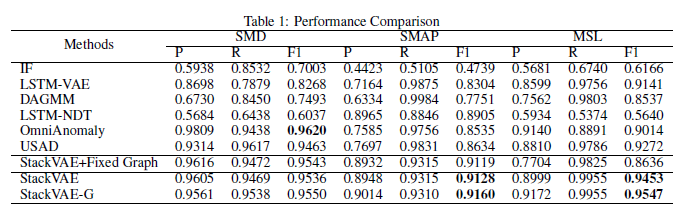Compared to OmniAnomaly, USAD, which has better results, the learning time is less than equal.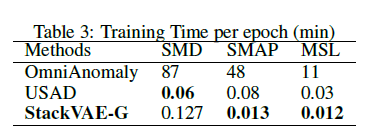### graph structure analysis

Lasso regression is used as the baseline for graph structure evaluation. Compared to the Lasso regression on the left of the figure below, StackVAE-G better shows the similarity between the channels, which is also confirmed by the real data.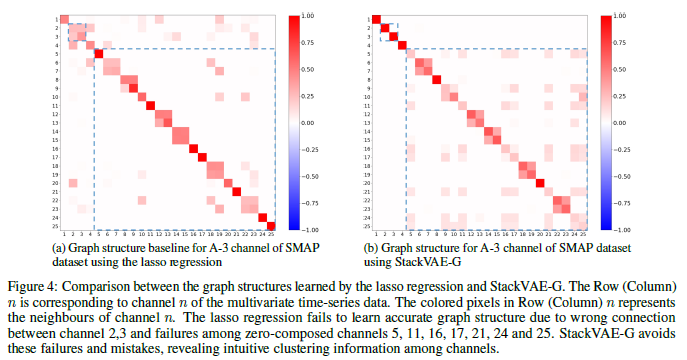As can be seen in the following figure, the graph structure serves to capture these similarity properties.